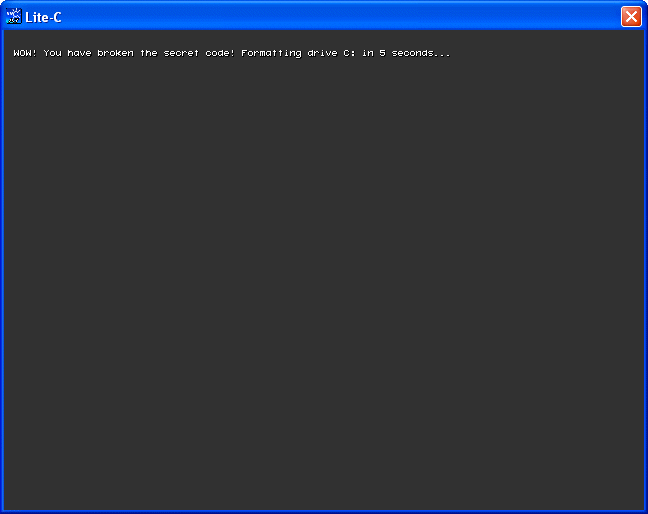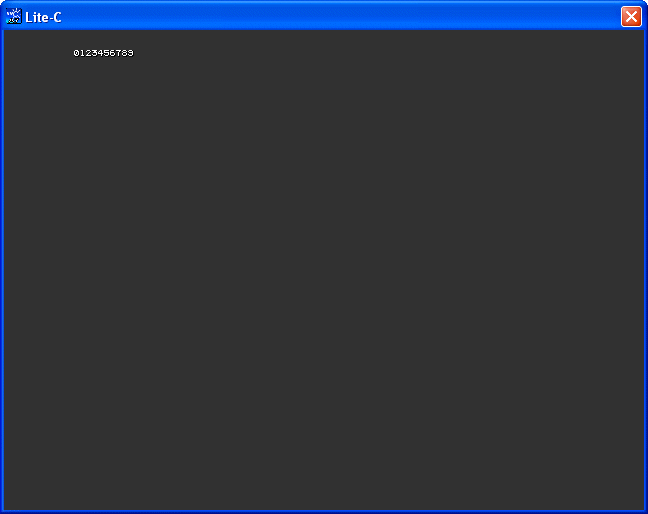Workshops
 This time we are going to explore 3 new, lite-C exclusive features. Start lite-C (or Sed) and then open the dowhile.c file from inside the litec103 folder.   #include  #include    float tax, vat = 0.15; // VAT = 15% in this example   STRING* amount_str = "      "; // accepts amounts up to 6 digits STRING* tax_str = "         "; STRING* temp_str = "         ";   TEXT* amount_txt =         {        layer = 15;        pos_x = 100;        pos_y = 20;        string (amount_str);        flags = VISIBLE; }    TEXT* tax_txt =         {        layer = 15;        pos_x = 250;        pos_y = 20;        string (tax_str);        flags = VISIBLE; }    function main() {        video_mode = 6; // create a program window of 640x480 pixels        vec_set(screen_color, vector(55, 55, 55)); // make the background color dark        do        {                str_cpy(amount_str, "      ");                inkey(amount_str);                tax = str_to_num(amount_str) * vat;                str_for_num(temp_str, tax);                str_cpy(tax_str, "Tax to pay: ");                str_cat(tax_str, temp_str);        }        while (!str_cmpi(amount_str, "0"));        sys_exit(NULL); }   Believe it or not, this program computes the VAT (the tax) that needs to be payed for a certain product value. Just type in a number and the program will compute 15% of its value (VAT is set to 0.15, but you can use any other value for it).The only part of the code that really needs our attention is the do-while loop:          do        {                str_cpy(amount_str, "      ");                inkey(amount_str);                tax = str_to_num(amount_str) * vat;                str_for_num(temp_str, tax);                str_cpy(tax_str, "Tax to pay: ");                str_cat(tax_str, temp_str);        }        while (!str_cmpi(amount_str, "0"));        sys_exit(NULL);   This particular loop has an interesting property: it repeats the instructions that are placed inside its brackets for as long as the expression that follows the "while" keyword is true. The loop stops when the expression, which in our case is (!str_cmpi(amount_str, "0")) becomes false. The do-while loops will run at least once because the expression is tested at the end of the loop.   Let's discuss the instructions from inside the brackets: the first line resets amount_str, placing the pointer at its starting position. We input the amount of money; it will be stored as a string inside amount_str. Then, we compute the tax by multiplying amount_str (which is converted from a string to a number using str_to_num) with the VAT value.   I could have used a panel with a digit in order to display the tax, but since the digits don't offer that much precision and I have used high precision (float) variables for tax and vat, I have decided to convert the numerical value back to a string and add it after the "Tax to pay: " string. All these instructions will be executed over and over until the player inputs "0" for the amount (amount_str is set to zero) - it makes sense to exit the program if that happens.   Another lite-C goodie is the "switch" instruction. I love the "if" instruction, but if you have to choose among several value, "switch" is way better. Don't take my word for it, see for yourself.   #include #include   int choice, input_value;   STRING* operation_str = "Press 1 for advice, 2 for an honest opinion or 3 for an insult";   TEXT* operation_txt =        {        layer = 15;        pos_x = 10;        pos_y = 20;        string (operation_str);        flags = VISIBLE; }   function main() {        video_mode = 6; // create a program window of 640x480 pixels        vec_set(screen_color, vector(55, 55, 55)); // make the background color dark        wait (-5); // display the text for 4 seconds        str_cpy(operation_str, " ");        inkey(operation_str);        input_value = str_to_num(operation_str);                                switch(input_value)        {                case 1:                        str_cpy(operation_str, "We can't give advice at the moment; try again later. Thanks for doing business with our company.");                        break;                case 2:                        str_cpy(operation_str, "We don't know you, but we think that you are doing a fabulous job!");                        break;                case 3:                        str_cpy(operation_str, "You, meat eater! And why are you laughing, you grass eater?");                        break;                default:                        str_cpy(operation_str, "WOW! You have broken the secret code! Formatting drive C: in 5 seconds...");        }                                wait (-5);        sys_exit(NULL); }   As you might have guessed, this is the code from inside the switch.c file. We display the text that's stored inside the operation_str string for 5 seconds, and then we allow the player to input the desired value (1, 2 or 3). Since we can't input numerical values directly, we use str_to_num to convert the input string to a number, and then we choose the proper "case" depending on the value of the number. Please note the "break" instructions; they make sure that only one of the "case" sections is executed. Finally, if the player types in a different value (4, 5 or maybe even a or z) the "default" section will be executed.Now try to imagine how you would choose among 10 different values using the "if" instruction, and then be thankful for the "switch" instruction, which allows us to add as many "case" sections as we want without complicating the code at all.   The last example is a bit more complicated, so fire up your synapses - we are going to discuss about recursive functions. These aren't quite new, but I didn't cover them in my previous workshops and they are very powerful. Let's open the recursive.c file and prepare for the worst!   #include #include   STRING* result_str = "          "; // stores up to 10 characters STRING* temp_str = " "; // stores 1 character   TEXT* result_txt =        {        layer = 15;        pos_x = 10;        pos_y = 20;        string (result_str);        flags = VISIBLE; }   function recurse(int i) {        if (i < 10)        {                str_for_num(temp_str, i); // let's convert i (a numerical value) to a string                str_cat(result_str, temp_str); // and then let's add the string to result_str                recurse(i + 1); // the function calls itself with a value equal with (i + 1) until i reaches 10        } }   function main() {        video_mode = 6; // create a program window of 640x480 pixels        vec_set(screen_color, vector(55, 55, 55)); // make the background color dark        recurse(0); // the argument of the function is set to zero at first }   I've scared you a bit, haven't I? As you see, our recursive function is very small; let's run the code and see what happens on the screen.Let me copy and paste the code for our recursive function right here:   function recurse(int i) {        if (i < 10)        {                str_for_num(temp_str, i); // let's convert i (a numerical value) to a string                str_cat(result_str, temp_str); // and then let's add the string to result_str                recurse(i + 1); // the function calls itself with a value equal with (i + 1) until i reaches 10        } }   And now let's see how it is called from inside function main( )          recurse(0);   The argument of the function is set to zero at first; this means that i will be set to zero as well. Since i is smaller than 10, the function will convert the numerical value to a string and add the result to result_str, displaying "0" on the screen. The last line inside the "if" parenthesis  will call the same function again, this time with an argument of i + 1 = 1, adding an "1" after our "0". The process will repeat for as long as i is smaller than 10; as soon as i reaches 10, "recurse(i + 1);" won't be executed anymore. Since the last value that worked ok was 9, the program will display 0123456789 on the screen, and then it will give control to the calling program.   Recursive functions set a new program control mechanism. Each one of them should have an "if" instruction; otherwise, the functions will run out of control until they use all the memory on the stack, freezing the computer. These functions are very useful; algorithms like Quicksort (one of the most powerful sorting algorithms) are very difficult to implement without using recursive functions. Don't worry, we will discuss more examples like this in the future.   Next month we will get to play with more shiny lite-C stuff so stay tuned!